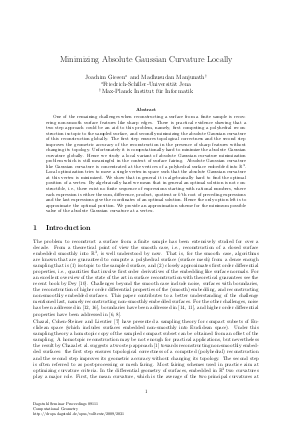Document# Minimizing Absolute Gaussian Curvature Locally

### Authors Joachim Giesen, Manjunath Madhusudan## File

DagSemProc.09111.3.pdf
• Filesize: 362 kB
• 16 pages

## Cite As

Joachim Giesen and Manjunath Madhusudan. Minimizing Absolute Gaussian Curvature Locally. In Computational Geometry. Dagstuhl Seminar Proceedings, Volume 9111, pp. 1-16, Schloss Dagstuhl - Leibniz-Zentrum für Informatik (2009)
https://doi.org/10.4230/DagSemProc.09111.3

## Abstract

One of the remaining challenges when reconstructing a surface from a finite sample is recovering non-smooth surface features like sharp edges. There is practical evidence showing that a two step approach could be an aid to this problem, namely, first computing a polyhedral reconstruction isotopic to the sampled surface, and secondly minimizing the absolute Gaussian curvature of this reconstruction globally. The first step ensures topological correctness and the second step improves the geometric accuracy of the reconstruction in the presence of sharp features without changing its topology. Unfortunately it is computationally hard to minimize the absolute Gaussian curvature globally. Hence we study a local variant of absolute Gaussian curvature minimization problem which is still meaningful in the context of surface fairing. Absolute Gaussian curvature like Gaussian curvature is concentrated at the vertices of a polyhedral surface embedded into \$mathbb{R}^3\$. Local optimization tries to move a single vertex in space such that the absolute Gaussian curvature at this vertex is minimized. We show that in general it is algebraically hard to find the optimal position of a vertex. By algebraically hard we mean that in general an optimal solution is not constructible, i.e., there exist no finite sequence of expressions starting with rational numbers, where each expression is either the sum, difference, product, quotient or \$k\$'th root of preceding expressions and the last expressions give the coordinates of an optimal solution. Hence the only option left is to approximate the optimal position. We provide an approximation scheme for the minimum possible value of the absolute Gaussian curvature at a vertex.
##### Keywords
• Absolute Gaussian curvature
• surface reconstruction
• mesh smoothing

## Metrics

• Access Statistics
• Total Accesses (updated on a weekly basis)
0